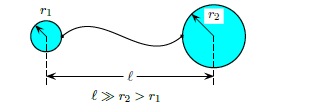# Problem: Two charged spherical conductors are connected by a long conducting wire. A total charge of q &gt; 0 is placed on this combination of two spheres. Sphere 1 has a radius of r1 and sphere 2 has a radius of r2, where r2 &gt; r1. If q1 represents the charge on sphere 1 and q 2 the charge on sphere 2, what is the ratio q1 / q2 of the charges? 1. q1 / q2 = r2 / r1 2. q1 / q2 = r1 / r1 + r2 3. q1 / q2 = (r2 / r1)2 4. q1 / q2 = r2 / r1 + r2 5. None of these 6. q1 / q2 = 1  7. q1 / q2 = (r1 / r2)2 8. q1 / q2 = r1 / r2

###### FREE Expert Solution
86% (296 ratings)
###### Problem Details

Two charged spherical conductors are connected by a long conducting wire. A total charge of q > 0 is placed on this combination of two spheres. Sphere 1 has a radius of r1 and sphere 2 has a radius of r2, where r2 > r1. If q1 represents the charge on sphere 1 and q 2 the charge on sphere 2, what is the ratio q1 / q2 of the charges?

1. q1 / q2 = r2 / r1

2. q1 / q2 = r1 / r1 + r2

3. q1 / q2 = (r2 / r1)2

4. q1 / q2 = r2 / r1 + r2

5. None of these

6. q1 / q2 = 1

7. q1 / q2 = (r1 / r2)2

8. q1 / q2 = r1 / r2Frequently Asked Questions

What scientific concept do you need to know in order to solve this problem?

Our tutors have indicated that to solve this problem you will need to apply the Electric Potential concept. You can view video lessons to learn Electric Potential. Or if you need more Electric Potential practice, you can also practice Electric Potential practice problems.

How long does this problem take to solve?

Our expert Physics tutor, Juan took 2 minutes and 13 seconds to solve this problem. You can follow their steps in the video explanation above.

What professor is this problem relevant for?

Based on our data, we think this problem is relevant for Professor Heidarian's class at UMD.